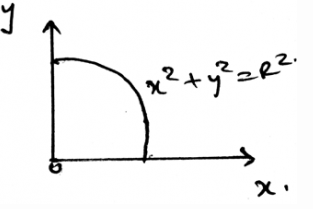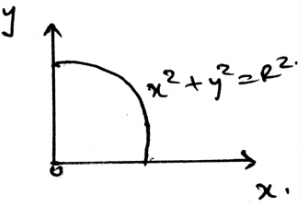Courses

# Test: Centre Of Mass

## 15 Questions MCQ Test Engineering Mechanics | Test: Centre Of Mass

Description
This mock test of Test: Centre Of Mass for Mechanical Engineering helps you for every Mechanical Engineering entrance exam. This contains 15 Multiple Choice Questions for Mechanical Engineering Test: Centre Of Mass (mcq) to study with solutions a complete question bank. The solved questions answers in this Test: Centre Of Mass quiz give you a good mix of easy questions and tough questions. Mechanical Engineering students definitely take this Test: Centre Of Mass exercise for a better result in the exam. You can find other Test: Centre Of Mass extra questions, long questions & short questions for Mechanical Engineering on EduRev as well by searching above.
QUESTION: 1

### The centre of mass is the ratio of ________ to _________

Solution:

The product of the centroid of the section to its mass to the total mass of the body is the centre of mass. Thus the answer. The ratio is generally used to locate the coordinate of the centroid or the centre of mass.

QUESTION: 2

Solution:
QUESTION: 3

### We use sometimes the measures to know the direction of moment in the calculations of the centre of mass. It is done by right handed coordinate system. Which is right about it(consider the mentioned axis to be positive)?

Solution:

As right handed coordinate system means that you are curling your fingers from positive x-axis towards y-axis and the thumb which is projected is pointed to the positive z-axis. Thus visualizing the same and knowing the basic members of axis will not create much problem.

QUESTION: 4

The total of all the masses of small particles adds up to give the total body mass. This mass lies along with gravity gives a force vector which is being passed by ________

Solution:

The total body mass along with the product of gravity always passes through the centre of gravity. All the forces are parallel is the right answer. Thus the total sum of all these small weights add up to the total weight of the body. Which passes through the centre of gravity.

QUESTION: 5

The all small masses that are being applied by all the infinite particles of the body act __________ to each other.

Solution:

All the small masses that are being applied by all the particles are being applied by them parallel to themselves. Thus the parallel is the right answer. Thus the total sum of all these small weights add up to the total weight of the body.

QUESTION: 6

A body’s all small particles have a small mass which is being applied by them to the body, which adds up to the total mass of the body.

Solution:

The weight of the body is the sum of the all the small masses that are been applied by all the particles. The smaller the particle the smaller the mass it applies. Thus the total mass of the body is the summation of all the infinite number of small masses applied to the body.

QUESTION: 7

What is not the condition for the equilibrium in calculations involved in the determination of the centre of mass?

Solution:

For the equilibrium in the three dimensional system of axis we have all the conditions true as, ∑Fx=0, ∑Fy=0 and ∑Fz=0. Also we have the summation of the forces equal to zero. Which is not a non-zero value.

QUESTION: 8

We first make equilibrium equations of the body by considering all the three dimensional forces and then the free body diagram is made and then equations are made to be applied so as to calculate the centre of mass.

Solution:

We first make the free body diagram and then we make the equilibrium equations to satisfy the given conditions. This helps us to solve the question easily. As this reduces the part of imagination and increases accuracy too.

QUESTION: 9

Determine the y coordinate of centroid of the wire in the shape of circle as shown.Solution:

The weight of the body is the sum of the all the small weights that are been applied by all the particles. The smaller the particle the smaller the weight it applies. Thus the total weight of the body is the summation of all the infinite number of small weights applied to the body.

QUESTION: 10

If solving the question in 3D calculations is difficult, then use the 2D system and then equate the ratio of the product of the centroid of the section to its mass to the total mass of the body to the centre of mass.

Solution:

The answer is obviously yes. If we are having any difficulty in making the vector components, then we can go in 2D. As if the particle is in equilibrium, the net force will be zero. No matter where you see first. Net force is zero.

QUESTION: 11

One of the use of the centre of mass or centroid is as in the simplification of the loading system the net force acts at the ___________ of the loading body.

Solution:

In the simplification of the loading system the net force acts at the centroid of the loading body. That is if the loading system is in the form of the triangle then the at the distance 2 by 3 of the base the net force of the loading will act. And the load will be half the area of the loading.

QUESTION: 12

If the non-Uniform loading is of the type of parabola then?

Solution:

The net force will act at the centroid of the parabola. Whether it be a parabola or the cubic curve the centroid is the only point at which the net force act. Force can’t be acted horizontally if the loading is vertical. Hence whatever be the shape of the loading, the centroid is the point of action of net force. Thus the use of centroid.

QUESTION: 13

If any external force also is applied on the distributed loading then?

Solution:

The external forces are treated differently. They are not added by the force of the distributed loading. That is the force not only acts at the centroid always. It can be shifted also. Depending on the external forces. Thus the use of centroid or centre of mass.

QUESTION: 14

The body is sometimes acted by two or three force members. The difference between the two and the three force members is ________________

Solution:

The definition of the two force member only defines that the forces are being acted on the two points on the body. So does is the definition of the three forces members. The points of action of the three forces are three.

QUESTION: 15

Determine the x coordinate of centroid of the wire in the shape of circle as shown.Solution:

The weight of the body is the sum of the all the small weights that are been applied by all the particles. The smaller the particle the smaller the weight it applies. Thus the total weight of the body is the summation of all the infinite number of small weights applied to the body.